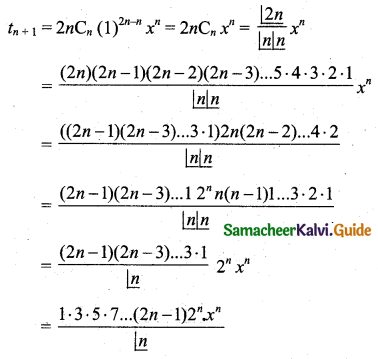Students can download 11th Business Maths Chapter 2 Algebra Ex 2.6 Questions and Answers, Notes, Samcheer Kalvi 11th Business Maths Guide Pdf helps you to revise the complete Tamilnadu State Board New Syllabus, helps students complete homework assignments and to score high marks in board exams.

## Tamilnadu Samacheer Kalvi 11th Business Maths Solutions Chapter 2 Algebra Ex 2.6

### Samacheer Kalvi 11th Business Maths Algebra Ex 2.6 Text Book Back Questions and Answers

Question 1.
Expand the following by using binomial theorem:
(i) (2a – 3b)4
(ii) $$\left(x+\frac{1}{y}\right)^{7}$$
(iii) $$\left(x+\frac{1}{x^{2}}\right)^{6}$$
Solution: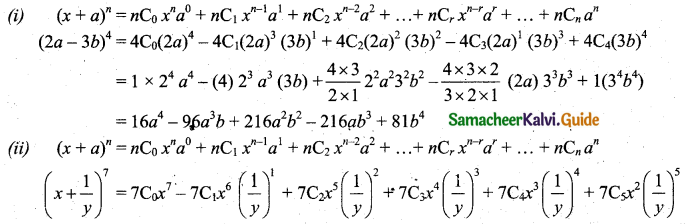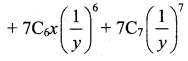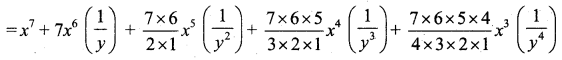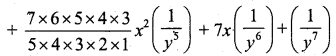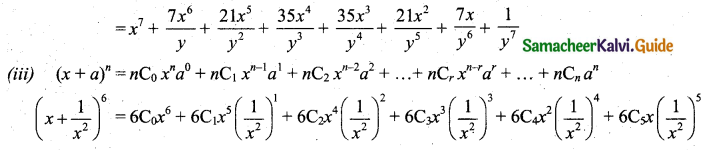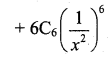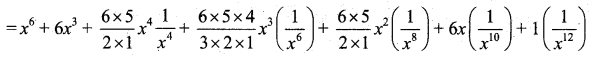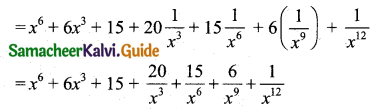Question 2.
Evaluate the following using binomial theorem:
(i) (101)4
(ii) (999)5
Solution:
(i) (x + a)n = nC0 xn a0 + nC1 xn-1 a1 + nC2 xn-2 a2 + ……… + nCr xn-r ar + …… + nCn an
(101)4 = (100 + 1)4 = 4C0 (100)4 + 4C1 (100)3 (1)1 + 4C2 (100)2 (1)2 + 4C3 (100)1 (1)3 + 4C4 (1)4
= 1 × (100000000) + 4 × (1000000) + 6 × (10000) + 4 × 100 + 1 × 1
= 100000000 + 4000000 + 60000 + 400 + 1
= 10,40,60,401

(ii) (x + a)n = nC0 xn a0 + nC1 xn-1 a1 + nC2 xn-2 a2 + ……… + nCr xn-r ar + …… + nCn an
(999)5 = (1000 – 1)5 = 5C0 (1000)5 – 5C1 (1000)4 (1)1 + 5C2 (1000)3 (1)2 – 5C3 (1000)2 (1)3 + 5C4 (1000)5 (1)4 – 5C5 (1)5
= 1(1000)5 – 5(1000)4 – 10(1000)3 – 10(1000)2 + 5(1000) – 1
= 1000000000000000 – 5000000000000 + 10000000000 – 10000000 + 5000 – 1
= 995009990004999Question 3.
Find the 5th term in the expansion of (x – 2y)13.
Solution:
General term is tr+1 = nCr xn-r ar
(x – 2y)13 = (x + (-2y))13
Here x is x, a is (-2y) and n = 13
5th term = t5 = t4+1 = 13C4 x13-4 (-2y)4
= 13C4 x9 24 y4
= $$\frac{13 \times 12 \times 11 \times 10}{4 \times 3 \times 2 \times 1}$$ × 2 × 2 × 2 × 2× x9y4
= 13 × 11 × 10 × 8x9y4
= 13 × 880x9y4
= 11440x9y4

Question 4.
Find the middle terms in the expansion of
(i) $$\left(x+\frac{1}{x}\right)^{11}$$
(ii) $$\left(3 x+\frac{x^{2}}{2}\right)^{8}$$
(iii) $$\left(2 x^{2}-\frac{3}{x^{3}}\right)^{10}$$
Solution:
(i) General term is tr+1 = nCr xn-r ar
Here x is x, a is $$\frac{1}{x}$$ and n = 11, which is odd.
So the middle terms are $$\frac{t_{n+1}}{2}=\frac{t_{11+1}}{2}, \frac{t_{n+3}}{2}=\frac{t_{11+3}}{2}$$
i.e. the middle terms are t6, t7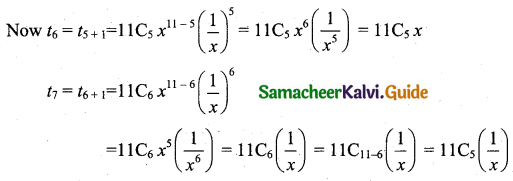(ii) Here x is 3x, a is $$\frac{x^{2}}{2}$$, n = 8, which is even.
∴ The only one middle term = $$\frac{t_{n+1}}{2}=\frac{t_{8+1}}{2}$$ = t5
General term tr+1 = nCr xn-r ar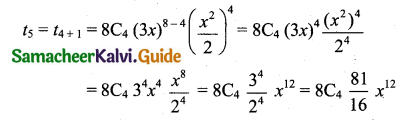(iii) $$\left(2 x^{2}-\frac{3}{x^{3}}\right)^{10}=\left(2 x^{2}+\frac{-3}{x^{3}}\right)^{10}$$ compare with the (x + a)n
Here x is 2x2, a is $$\frac{-3}{x^{3}}$$, n = 10, which is even.
So the only middle term is $$\frac{t_{n+1}}{2}=\frac{t_{10}}{2}+1$$ = t6
General term tr+1 = nCr xn-r ar
t6 = t5+1 = tr+1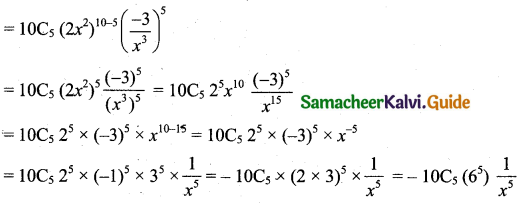Question 5.
Find the term in dependent of x in the expansion of
(i) $$\left(x^{2}-\frac{2}{3 x}\right)^{9}$$
(ii) $$\left(x-\frac{2}{x^{2}}\right)^{15}$$
(iii) $$\left(2 x^{2}+\frac{1}{x}\right)^{12}$$
Solution:
(i) Let the independent form of x occurs in the general term, tr+1 = nCr xn-r ar
Here x is x2, a is $$\frac{-2}{3 x}$$ and n = 9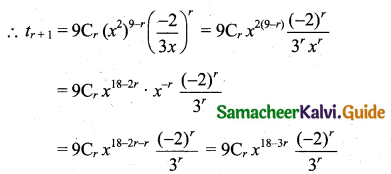Independent term occurs only when x power is zero.
18 – 3r = 0
⇒ 18 = 3r
⇒ r = 6
Put r = 6 in (1) we get the independent term as 9C6 x0 $$\frac{(-2)^{6}}{3^{6}}$$ = 9C3 $$\left(\frac{2}{3}\right)^{6}$$
[∵ 9C6 = 9C9-6 = 9C3]

(ii) $$\left(x-\frac{2}{x^{2}}\right)^{15}=\left(x+\frac{-2}{x^{2}}\right)^{15}$$ compare with the (x + a)n
Here x is x, a is $$\frac{-2}{x^{2}}$$, n = 15.
Let the independent term of x occurs in the general term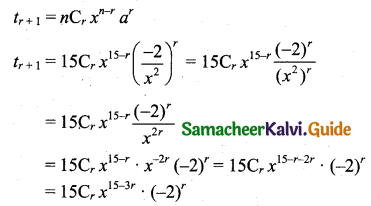Independent term occurs only when x power is zero.
15 – 3r = 0
15 = 3r
r = 5
Using r = 5 in (1) we get the independent term
= 15C5 x0 (-2)5 [∵ (-2)5 = (-1)5 25 = -25]
= -32(15C5)

(iii) $$\left(2 x^{2}+\frac{1}{x}\right)^{12}$$ Compare with the (x + a)n.
Here x is 2x2, a is $$\frac{1}{x}$$, n = 12.
Let the independent term of x occurs in the general term.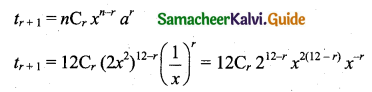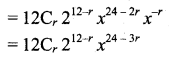Independent term occurs only when x power is zero
24 – 3r = 0
24 = 3r
r = 8
Put r = 8 in (1) we get the independent term as
= 12C8 212-8 x0
= 12C4 × 24 × 1
= 7920

Question 6.
Prove that the term independent of x in the expansion of $$\left(x+\frac{1}{x}\right)^{2 n}$$ is $$\frac{1 \cdot 3 \cdot 5 \cdot \cdot \cdot(2 n-1) 2^{n}}{n !}$$
Solution:
There are (2n + 1) terms in expansion.
∴ tn+1 is the middle term.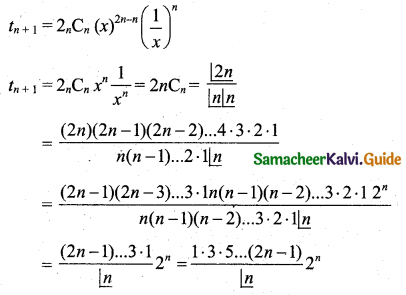Question 7.
Show that the middle term in the expansion of is (1 + x)2n is $$\frac{1 \cdot 3 \cdot 5 \ldots(2 n-1) 2^{n} x^{n}}{n !}$$
Solution:
There are 2n + 1 terms in expansion of (1 + x)2n.
∴ The middle term is tn+1.